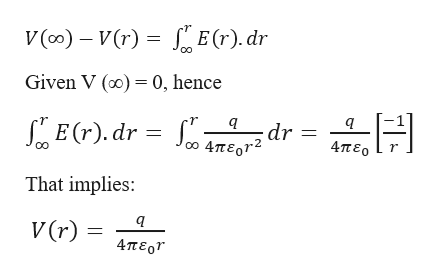Question

Part B through F Please. I have no idea where to start.

metal sphere with radius ra is supported on an insulating stand at the center of a hollow, metal, spherical shell with radius rb. There is charge
+q on the inner sphere and charge −q on the outer spherical shell. Take
V to be zero when is infinite.

Part B
Calculate the potential V(r) for ra<r<rb.
Express your answer in terms of the given quantities and appropriate constants.

Part C
Calculate the potential V(r)for r>rb.
Express your answer in terms of the given quantities and appropriate constants.

Part D
Find the potential of the inner sphere with respect to the outer.
Express your answer in terms of the given quantities and appropriate constants

Part E
Use the equation Er=−∂V/∂r and the result from part (b) to find the electric field at any point between the spheres (ra<r<rb).
Express your answer in terms of the given quantities and appropriate constants.

Part F
Use the equation Er=−∂V/∂r and the result from part (c) to find the electric field at a point outside the larger sphere at a distance r from the center, where r>rb
Express your answer in terms of the given quantities and appropriate constants.

check_circleExpert Solution
Step 1

Given information:

Charge on the inner sphere = +q

Radius of the inner sphere = ra

Charge on the outer spherical shell = -q

Radius of the outer shell = rb

Step 2

Electric potential at r where, ra < r < rb

The equation of electric potential in terms of electric field is given as:

Step 3

Part B:

The electric potential...help_outlineImage TranscriptioncloseE(r). dr V (co) V(r) Given V () 0, hence E(r). dr dr = 4περ 0 4περr2 That implies: V (r) 4περ fullscreen

### Want to see the full answer?

See Solution

#### Want to see this answer and more?

Solutions are written by subject experts who are available 24/7. Questions are typically answered within 1 hour*

See Solution
*Response times may vary by subject and question
Tagged in

### Physics